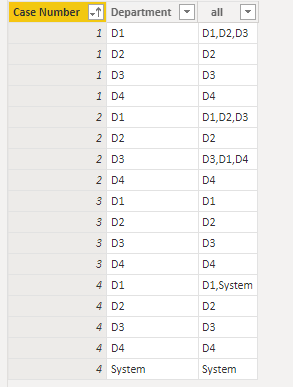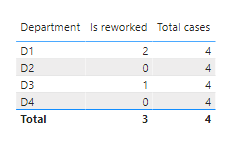cancel
Showing results for
Did you mean:Helper III

## Calculating the rework using previous department

Need a quick help from the communty.

below is the raw data. highlighted are the rework cases

 Case Number Department Out time 1 D1 1 1 D2 2 1 D2 3 1 D2 4 1 D3 5 1 D3 6 1 D1 7 1 D1 8 1 D4 9 1 D4 10 2 D1 11 2 D2 12 2 D2 13 2 D2 14 2 D3 15 2 D3 16 2 D1 17 2 D1 18 2 D4 19 2 D4 20 2 D3 21 3 D1 22 3 D2 23 3 D3 24 3 D4 25 4 D1 26 4 System 27 4 D1 28 4 D2 29 4 D3 30 4 D4 31

We need to create a measure based on the condition if the same Department is tagged again to the same from a new previous department then it will marked as rework. Need to ignore if the department is System.

Final Output

 Department Number of reworks Total Cases D1 2 4 D2 0 4 D3 1 4 D4 0 4

1 ACCEPTED SOLUTIONCommunity Support

My solution is as below. I create a new table to help the calculation. (I add an Index column to the raw table with Power Query Editor. If your "Out time" column has the same meaning, you can use it instead.)

``````Table 2 = SUMMARIZE('Table','Table'[Case Number],'Table'[Department],"all",
var minIndex = MIN('Table'[Index])
var maxIndex = MAX('Table'[Index])
var departmentTable = CALCULATETABLE(VALUES('Table'[Department]),ALLEXCEPT('Table','Table'[Case Number]),'Table'[Index]>=minIndex,'Table'[Index]<=maxIndex)
return
CONCATENATEX(departmentTable,'Table'[Department],","))``````According to above table, if "all" column has other values except "System" and the Department value on the same row, it means this Department is tagged again for the case. So create a new column

``Is reworked = IF(NOT(CONTAINSSTRING('Table 2'[all],",")), 0, IF('Table 2'[all] IN {"System"&","&'Table 2'[Department], 'Table 2'[Department]&","&"System"}, 0, 1))``OutputBest Regards,
Community Support Team _ Jing
If this post helps, please Accept it as Solution to help other members find it.Community Support

My solution is as below. I create a new table to help the calculation. (I add an Index column to the raw table with Power Query Editor. If your "Out time" column has the same meaning, you can use it instead.)

``````Table 2 = SUMMARIZE('Table','Table'[Case Number],'Table'[Department],"all",
var minIndex = MIN('Table'[Index])
var maxIndex = MAX('Table'[Index])
var departmentTable = CALCULATETABLE(VALUES('Table'[Department]),ALLEXCEPT('Table','Table'[Case Number]),'Table'[Index]>=minIndex,'Table'[Index]<=maxIndex)
return
CONCATENATEX(departmentTable,'Table'[Department],","))``````According to above table, if "all" column has other values except "System" and the Department value on the same row, it means this Department is tagged again for the case. So create a new column

``Is reworked = IF(NOT(CONTAINSSTRING('Table 2'[all],",")), 0, IF('Table 2'[all] IN {"System"&","&'Table 2'[Department], 'Table 2'[Department]&","&"System"}, 0, 1))``OutputBest Regards,
Community Support Team _ Jing
If this post helps, please Accept it as Solution to help other members find it.